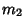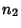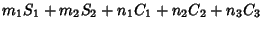Simplicity

The number of operations needed to effect a Geometric Construction as determined in Geometrography. If the number of operations of the five Geometrographic types are denoted,,,, and, respectively, then the simplicity isand the symbol. It is apparently an unsolved problem to determine if a given Geometric Construction is of smallest possible simplicity.